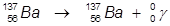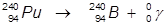# Gamma Decay

One of the three main types of radioactive decay is known as gamma decay (γ-decay). During gamma decay, the energy of the parent atom is changed by the emission of a photon. The resulting energy of the daughter atom is lower than the parent atom.

A photon is a massless particle with a very small wavelength. The energy of the photon is large and therefore has a large penetration effect. This could lead to serious problems for biological material, such as human tissue.

Examples:

1. The γ-decay of barium-137.In this example, the parent atom is lowered in energy.

2. The γ-decay of plutonium-240.In this example, the parent atom is lowered in energy.

 Related Links: Chemistry Nuclear Chemistry Alpha Decay Beta Decay Nuclear Fusion Nuclear Reactions

To link to this Gamma Decay page, copy the following code to your site: